Draw it!

Draw two lines c, d that c || d. On line c mark the points A, B.
By point A lead perpendicular line to c. By point B lead perpendicular line to c.

Result

x=##:  0

Leave us a comment of example and its solution (i.e. if it is still somewhat unclear...):Be the first to comment!Next similar examples:

1. Straight lines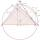Draw two lines c, d so that c || d. On line c mark points A, B, from point A start perpendicular to line c, from point B perpendicular to line c.
2. Three points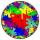Mark three points E, F and G in the plane not lie on one line. a) Draw a line segment FG b) Construct halfline (ray) EG c) Draw a line EF
3. Line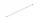How many parts of line divide 5 (different) points that lie on it?
4. Flood waterFlood waters in some US village meant that the homes had to evacuate 364 people. 50 of them stayed at elementary schools, 59 them slept with their friends and others went to relatives. How many people have gone to relatives?
5. CakesOn the bowl were a few cakes. Jane ate one-third of them, Dana ate a quarter of those cakes that remained. a) What part (of the original number of cakes) Dana ate? b) At least how many cakes could be (initially) on thebowl?
6. Neighbor angle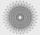For 136° angle calculate size of adjacent angle on one side of a straight line.
7. Plane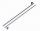On how many parts divide plane 6 parallels?
8. Angles 1It is true neighboring angles have not common arm?
9. One frame5 picture frames cost € 12 more than three frames. How much cost one frame?
10. Isosceles trapezium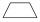Trapezoid YSED (YS||ED) is isosceles. The size of the angle at vertex Y is 17 degrees. Calculate the size of the angle at vertex E.
11. Angles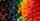Which of those angles are obtuse?Added together and write as decimal number: LXVII + MLXIV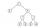Find out value of expressions if a = -1, b =2: x=b - 2a - ab y=a3 - b2 - 2ab z=a2 b3 - a3 b2 w=a + b + a3 - b2How many times I decrease the number 1632 to get the result 24?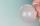10 pupils went to the store. 6 pupils bought lollipops and 9 pupils bought chewing gum. How many pupils have bought both lollipops and chewing gums (if everyone bought something)?If 5x - 17 = -x + 7, then x =Honza had three cages (black, silver, gold) and three animals (guinea pig, rat and puppy). There was one animal in each cage. The golden cage stood to the left of the black cage. The silver cage stood on the right of the guinea pig cage. The rat was in the